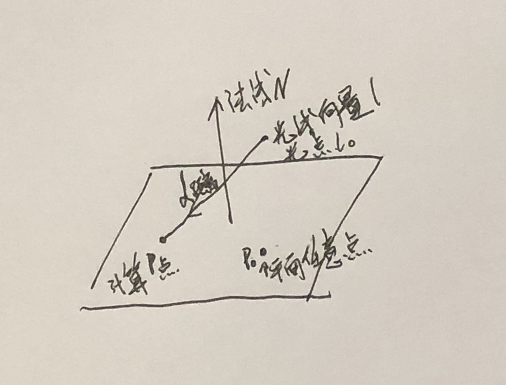## 《Unity3D高级编程之进阶主程》第七章，渲染管线与图形学(五) - Projector投影原理

### Projector 投影的原理与应用

``````
sampler2D _MainTex;
float4x4 unity_Projector;
struct v2f{
float4 pos:SV_POSITION;
float4 texc:TEXCOORD0;
}

v2f vert(appdata_base v)
{
v2f o;
o.pos = mul(UNITY_MAXTRIX_MVP, v.vertex);
o.texc = mul(unity_Projector, v.vertex);
return o;
}

float4 frag(v2f i) : COLOR
{
float4 c = tex2Dproj(_MainTex, i.texc);
return c;
}

``````

``````	tex2Dproj(texture,uvproj);

这个纹理投影函数，其实就是在使用之前会将该投影纹理坐标除以透视值

可以等价于按如下方法使用普通二维纹理查询函数

float4 uvproj = uvproj/uvproj.w;

tex2D(texture,uvproj);
``````

``````缺图
``````

​###### 投影的技巧还有很多，我们下面介绍几种投影技巧在游戏项目中的运用。

###### 平面阴影

``````	缺图
``````

``````	(p - TerrainPos) 点乘 TerrainNormal = 0
``````

``````	p = d*L + L0 其中L0为顶点，的l为光到顶点的射线方向，d为L0射到p点的距离。
``````

``````	(dL + L0 - TerrainPos) 点乘 TerrainNormal = 0
``````

``````	dL 点乘 TerrainNormal + (L0 - TerrainPos) 点乘 TerrainNormal = 0
``````

``````	d = ((TerrainPos - L0) 点乘 TerrainNormal) / (l 点乘 TerrainNormal)
``````

``````Pass
{
CGPROGRAM

#pragma vertex vert
#pragma fragment frag
#include "UnityCG.cginc"

struct appdata
{
float4 vertex : POSITION;
};

struct v2f
{
float4 vertex : SV_POSITION;
};

float4 TerrainPos, TerrainNormal;
v2f vert (appdata v)
{
v2f o;
float4 wPos = mul(unity_ObjectToWorld, v.vertex);
// 光的方向
float3 direction = normalize(_WorldSpaceLightPos0.xyz);
// d 值的计算
float dist = dot(TerrainPos.xyz - wPos.xyz, TerrainNormal.xyz) / dot(direction, TerrainNormal.xyz);
// 代入 p = d * l + L0 公式
wPos.xyz = wPos.xyz + dist * direction;
// 空间顶点转换
o.vertex = mul(unity_MatrixVP, wPos);
return o;
}

fixed4 frag (v2f i) : SV_Target
{
return fixed4(0,0,0,1);
}
ENDCG
}
``````###### 利用深度信息计算图片投影

``````	绘制一个box，其纹理的展示方式则以深度信息为依据，纹理贴近其绘制的模型上。
``````

###### 贴花(喷图)

《维基百科 Line–plane intersection》 https://en.wikipedia.org/wiki/Line%E2%80%93plane_intersection

· 书籍著作, Unity3D, 前端技术

• 版权申明

本文为博主原创文章，未经允许不得转载:

《Unity3D高级编程之进阶主程》第七章，渲染管线与图形学(五) - Projector投影原理

•